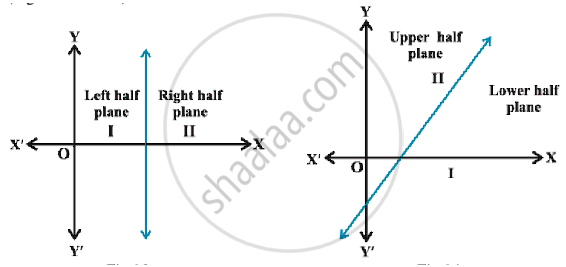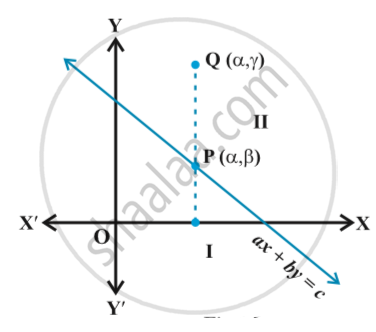Advertisement Remove all ads

# Graphical Solution of Linear Inequalities in Two Variables

Advertisement Remove all ads

#### description

Linear Inequalities - Graphical Representation of Linear Inequalities in Two Variables

#### notes

We know that a line divides the Cartesian plane into two parts. Each part is known as a half plane. A vertical line will divide the plane in left and right half planes and a non-vertical line will divide the plane into lower and upper half planes Fig.A point in the Cartesian plane will either lie on a line or will lie in either of the half planes I or II. We shall now examine the relationship, if any, of the points in the plane and the inequalities ax + by < c or ax + by > c.
Let us consider the line
ax + by = c, a ≠ 0, b  ≠ 0 ... (1)
There are three possibilities namely:
(i) ax + by =  c (ii) ax + by > c (iii) ax + by < c.
In case (i), clearly, all points (x, y) satisfying (i) lie on the line it represents and conversely. Consider case (ii), let us first assume that b > 0. Consider a point P (α,β) on the line ax + by = c, b > 0, so that aα + bβ = c.Take an arbitrary point Q (α , γ) in the half plane II . figNow ,  we interpret, γ > β  (Why?)
or bγ > bβ    or    aα + b γ > aα + bβ (Why?)
or aα + b γ > c i.e., Q(α,γ ) satisfies the inequality ax + by > c.
Thus, all the points lying in the half plane II above the line ax + by = c satisfies the inequality ax + by > c. Conversely, let (α, β) be a point on line ax + by = c and an arbitrary point Q(α, γ) satisfying
ax + by > c
so that    aα + bγ > c
⇒ aα + b γ > aα + bβ (Why?)
⇒ γ > β (as b > 0)
This means that the point (α, γ) lies in the half plane II.
Thus, any point in the half plane II satisfies ax + by > c, and conversely any point satisfying the inequality ax + by > c lies in half plane II.
In case b < 0, we can similarly prove that any point satisfying ax + by > c lies in the half plane I, and conversely.
Hence, we deduce that all  points satisfying ax + by > c lies in one of the half planes II or I according as b > 0 or b < 0, and conversely.
Thus, graph of the inequality ax + by > c will be one of the half plane (called solution region) and represented by shading in the corresponding half plane.

If you would like to contribute notes or other learning material, please submit them using the button below.

### Shaalaa.com

Graphical Solution of Linear Inequalities in Two Variables [00:10:56]
S
##### Series:
0%

Advertisement Remove all ads
Share
Notifications

View all notifications

Forgot password?